# Example Code

## Products and Environment

This section reflects the products and operating system used to create the example.

### Software

• LabVIEW MathScript RT Module
• LabVIEW
• LabVIEW MathScript RT Module

## Overview

LabVIEW MATLAB Script nodes are recommended as an alternative to LabVIEW MathScript nodes for Windows computers. MATLAB Script nodes can be used for a wide variety of tasks including data analysis, curve fitting, spectral analysis, signal processing, statistics, and machine learning. Graphical LabVIEW code can be combined with MATLAB Script nodes and used for system programming, automation, and I/O.

This document describes the differences between the MATLAB Script node and MathScript node in LabVIEW, shows both desktop and real-time options for moving away from MathScript nodes, and also describes example benchmark VIs for several computational tasks. If you are interested in examples of how to accomplish the same mathematical task in different ways with LabVIEW, you can review this example: Three Ways to do Mathematical Analysis in LabVIEW

## Description

Key differences between MATLAB Script nodes and MathScript nodes

The attached example benchmark VIs include both MATLAB Script nodes and MathScript nodes. The following table highlights some key differences between the nodes. MATLAB Script nodes make ActiveX calls to MATLAB software installed on the same machine to execute scripts. You must have licensed copies of both LabVIEW and MATLAB on your Windows computer in order to use MATLAB Script nodes in LabVIEW. There are several benefits to this approach. You will have full access to all the toolboxes, objects, and data types included in your MATLAB license. You will also have full syntax and numerical compatibility with MATLAB.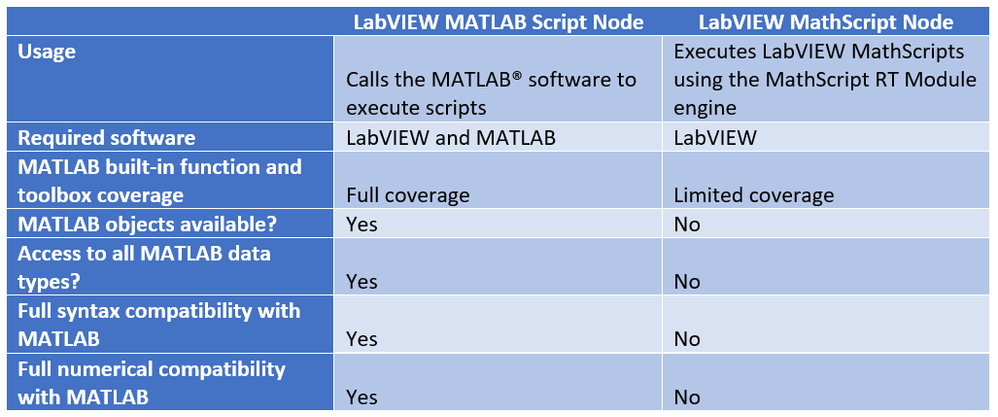Key differences between MATLAB Script nodes and MathScript nodes

Options for migration to either IDE-IDE communication methods with MATLAB or to compiled MATLAB code

The decision tree below highlights some additional options for migration away from MathScript nodes and towards either IDE to IDE communication methods with MATLAB or towards compiled code options developed with MathWorks code compilation software. You should first review your application to determine if it is a desktop application or if it is also leveraging MathScript on real-time targets. If it is a desktop application, then you can move from MathScript nodes to MATLAB Script nodes in LabVIEW or you can make the switch to LabVIEW NXG and the Interface for MATLAB. If you are considering LabVIEW NXG, make sure that the rest of your application is ready to port as well and that your hardware is fully supported.

The decision tree also describes options for LabVIEW Real-Time applications and for Mac/Linux desktop computers. For LabVIEW Real-Time applications, you should consider the Model Interface Toolkit combined with compiled MATLAB code as an alternative to the MathScript node.  You can take your script and put it in a MATLAB Function block in a Simulink diagram. The Simulink model can consist primarily of one or more MATLAB function blocks along with inports and outports.  If your Simulink model does not include any continuous-time components, then, instead of choosing a continuous-time solver, you can choose a fixed-step discrete solver for your real-time application. The Running Compiled Simulink® Models in LabVIEW and in VeriStand document includes an example with a MATLAB Function block inside of a Simulink diagram.

If you have a real-time application but do not have access to Simulink and Simulink Coder, then you can use a Call Library Function node in LabVIEW Real-Time along with your compiled MATLAB code.

For Mac or Linux desktop computers, you can choose to use the System Exec VI for IDE to IDE communication with a licensed copy of MATLAB or you can use a Call Library Function node with compiled MATLAB code.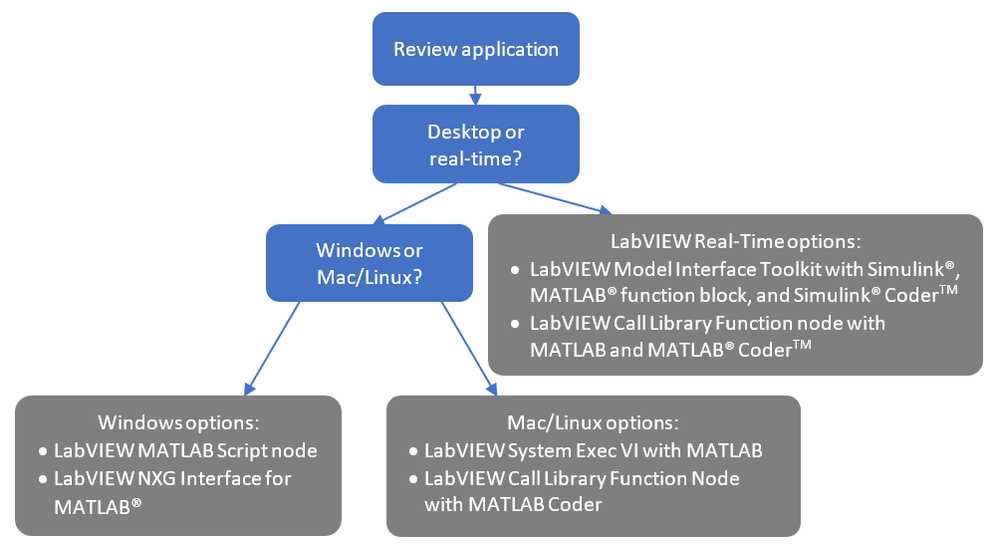Example benchmark VIs for MATLAB Script nodes and MathScript nodes

Three VIs are attached to this document and they include example benchmarks for MATLAB Script nodes and MathScript nodes along with graphical LabVIEW code.  Each of the approaches is included as a separate case in a case structure.  The three VIs include the following tasks:

• Fast Fourier transform (FFT) of an input array
• Lower upper (LU) factorization of an input matrix
• Numerical solution of an ordinary differential equation (ODE) specified in an example script (secondord.m)

These example VIs run the calculations several times and compute the average calculation time. You can specify the number of calculations to run along with the number of warmup calculations to exclude.  The option to exclude warmup calculations was created in consideration of the initiation of the communication with MATLAB for the MATLAB Script node.

Both the FFT and LU example benchmark VIs utilize the same input array and matrix respectively for each method.  The ODE example benchmark VI uses the same differential equation and initial conditions for each method.

Although the underlying computational engines are different for the MATLAB Script node and MathScript nodes, the settings for each method are chosen to provide similar numerical results. One note on the ODE benchmark is that the MATLAB Script node does not allow the user to choose a minimum step time for the variable step solver. A check on the number of points for the MATLAB solution showed a slightly higher number of points compared to MathScript, 121 versus 112 respectively, but the average time to calculate the MATLAB solution was still less than MathScript.

The table below shows the results of running the three VIs on a Windows computer.  For each VI, the number of runs chosen was 20 along with the exclusion of 2 warmup runs from the average calculation time for each method. The MATLAB Script node is faster than the MathScript node for both the LU and ODE tasks. It is slower for the FFT task.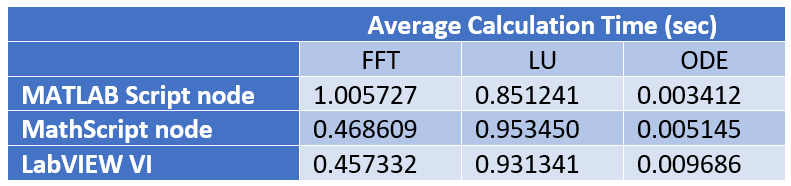Windows benchmarks results table, 20 runs with 2 warmup runs excluded

(Intel Core i7-7600U CPU @ 2.80GHz)

You can modify the example benchmark VIs with your own code and appropriate inputs in order to calculate custom benchmarks for your application.

Outputs from each node or the graphical code were not included on the example benchmark VIs. You can copy the code in the cases to new VIs to examine and compare outputs. The response plots for the ODE calculations are included in additional examples in a separate zip file attached to this document.

Screenshots for the VIs and the secondord.m script are shown below.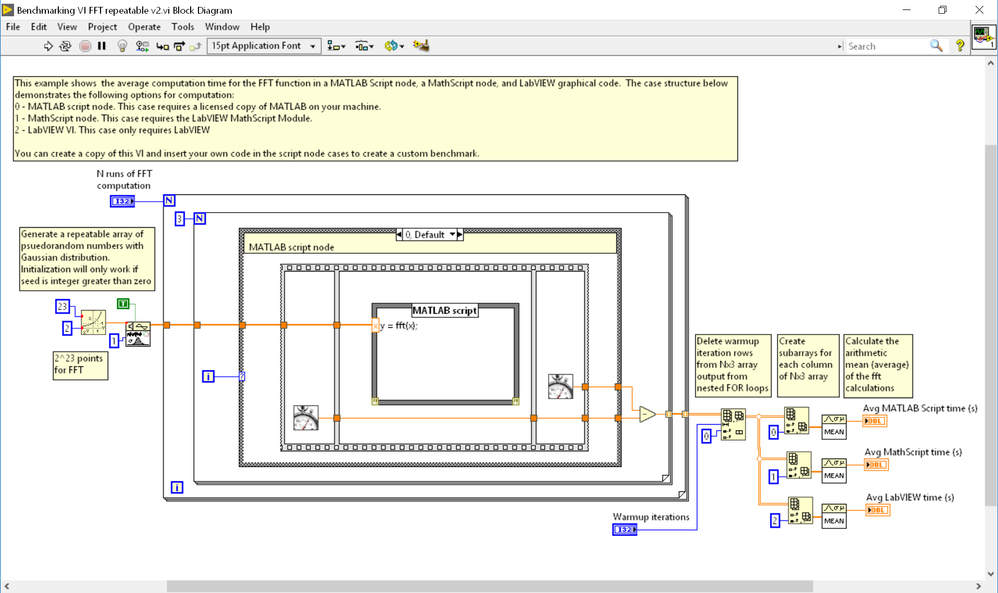Screenshot for FFT example benchmark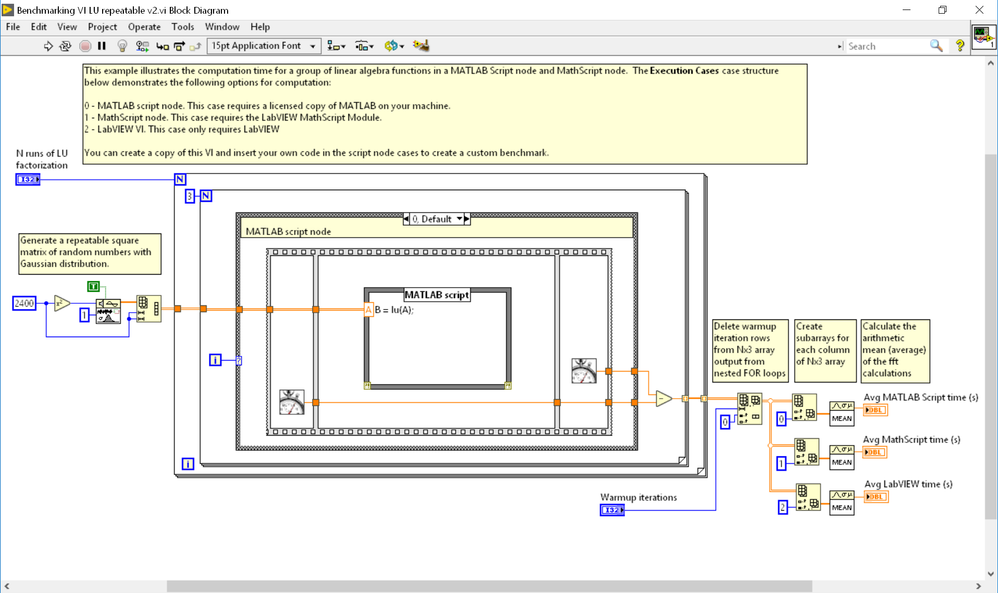Screenshot for LU example benchmark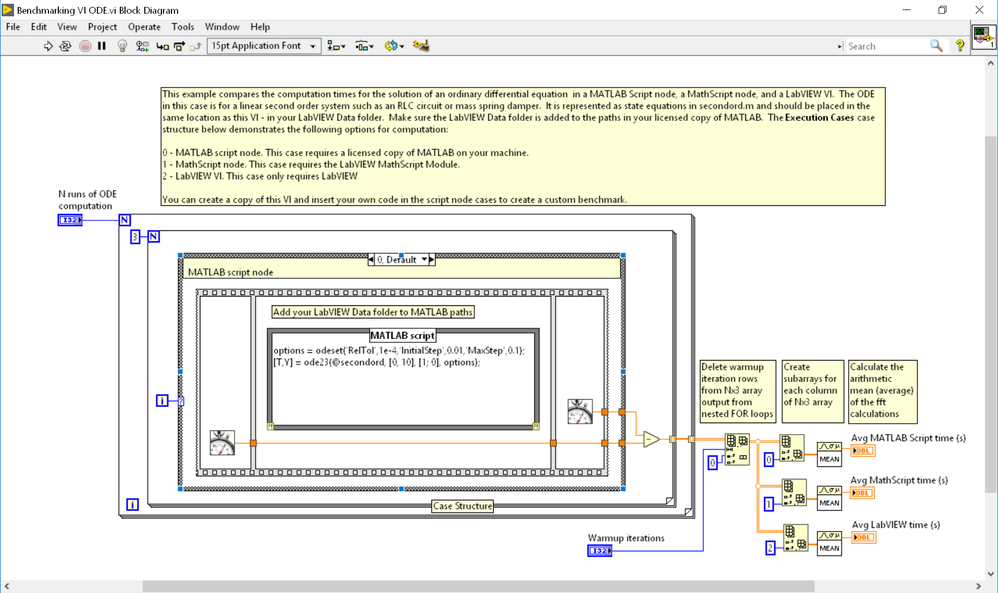Screenshot for ODE example benchmark

Secondord.m:

function dydt = secondord(t, y);

dydt = [y(2); -2*y(1) - y(2)];

## Hardware and Software Requirements

Required software for Windows:

• LabVIEW 2018 (32-bit) or later
• LabVIEW 2018 MathScript Module (32-bit) or later
• MATLAB 2018a or later

No hardware required.

## Steps to Implement or Execute Code

Installing the examples and setting the path in MATLAB:

Place the following three example benchmark VIs and supporting script (from the Example Benchmarks for MATLAB Script and MathScript zip file) into the Documents > LabVIEW Data folder on your Windows computer:

• Benchmarking FFT VI
• Benchmarking LU VI
• Benchmarking ODE VI
• secondord.m

Also make sure that you add the path for the LabVIEW Data folder to your MATLAB environment on the same machine.

Running the example benchmark VIs:

You can run each example benchmark VI and observe the results. If desired, you can change the number of runs and the warmup runs to exclude from the average time for each calculation.

Acknowledgements:

----------------------------------------------

Jeannie Sullivan Falcon, Ph.D.

Principal Engineer, Space Avionics Dept.

Space Science & Engineering Division

Southwest Research Institute

----------------------------------------------

Example code from the Example Code Exchange in the NI Community is licensed with the MIT license.Member
on

You may find Matlab script nodes are slow (and even unreliable) if you perform data transfer of large arrays.  You may find it's better to save the data to a file, and call a Matlab function to read the data file, and then process it.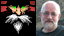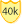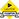Knight of NI
on

It seems absolutely pointless to use scripts with a single slow function, washing out all the potential differences in calling overhead. It would be much more interesting to do a long script with basic calculations (Matlab script, Mathscript) and compare with the same using LabVIEW structures and primitives).

It always hurts the eye to see multiple unneeded coercion dots (example) and single-output index array function instead of a properly resized copy: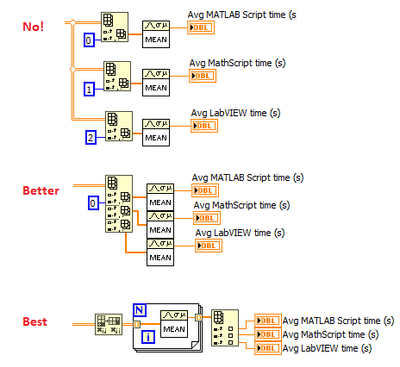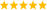LabVIEW Champion. It all comes together inGCentralWhat does "Engineering Redefined" mean??
Contributors